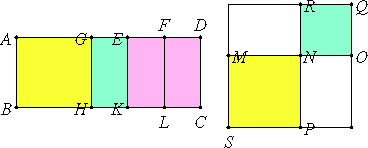# Proposition 57

If an area is contained by a rational straight line and the fourth binomial, then the side of the area is the irrational straight line called major.

Let the area AC be contained by the rational straight line AB and the fourth binomial AD divided into its terms at E, of which terms let AE be the greater.

I say that the side of the area AC is the irrational straight line called major.

X.Def.II.4

Since AD is a fourth binomial straight line, therefore AE and ED are rational straight lines commensurable in square only, the square on AE is greater than the square on ED by the square on a straight line incommensurable with AE, and AE is commensurable in length with AB.X.18

Bisect DE at F, and apply to AE a parallelogram, the rectangle AG by GE, equal to the square on EF. Then AG is incommensurable in length with GE.

Draw GH, EK, and FL parallel to AB, and make the rest of the construction as before.

It is then manifest that MO is the side of the area AC.

It is next to be proved that MO is the irrational straight line called major.

Since AG is incommensurable with EG, therefore AH is also incommensurable with GK, that is, SN with NQ. Therefore MN and NO are incommensurable in square.

X.19

Since AE is commensurable with AB, therefore AK is rational, and it equals the sum of the squares on MN and NO. Therefore the sum of the squares on MN and NO is also rational.

X.13

Since DE is incommensurable in length with AB, that is, with EK, while DE is commensurable with EF, therefore EF is incommensurable in length with EK.

X.21

Therefore EK and EF are rational straight lines commensurable in square only. Therefore LE, that is, MR, is medial.

And it is contained by MN and NO, therefore the rectangle MN by NO is medial. And the sum of the squares on MN and NO is rational, and MN and NO are incommensurable in square.

X.39

But, if two straight lines incommensurable in square and making the sum of the squares on them rational, but the rectangle contained by them medial, are added together, then the whole is irrational and is called major. Therefore MO is the irrational straight line called major and is the side of the area AC.

Therefore, if an area is contained by a rational straight line and the fourth binomial, then the side of the area is the irrational straight line called major.

Q.E.D.

## Guide

This proposition is used in X.70.